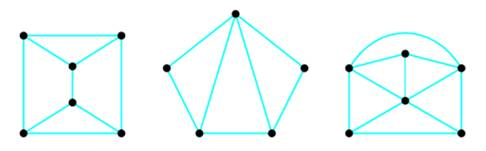# Dual Graph Every planar graph has what is called a dual graph. To form the dual graph, start with a planar drawing of the graph. With a different-color pen, draw a dot in each face (including the infinite face). These will be the vertices of the dual graph. Now, for every edge in the original graph, draw a new edge that crosses over it and connects the two new vertices in the faces on each side of the original edge. The resulting dual graph is always itself a planar graph. (Note that it may contain multiple edges.) The procedure is illustrated below. Draw the dual graph of each of the planar graphs below. For each of the graphs in part a, count the number of faces, edges, and vertices in the original graph and compare your results with the numbers in the dual graph. What do you notice? Explain why this will always be true. Start with your dual graph of the first graph in part a, and find its dual. What do you notice?### Mathematical Excursions (MindTap C...

4th Edition
Richard N. Aufmann + 3 others
Publisher: Cengage Learning
ISBN: 9781305965584

#### Solutions

Chapter
Section### Mathematical Excursions (MindTap C...

4th Edition
Richard N. Aufmann + 3 others
Publisher: Cengage Learning
ISBN: 9781305965584
Chapter 5.3, Problem 30ES
Textbook Problem
57 views

## Dual Graph Every planar graph has what is called a dual graph. To form the dual graph, start with a planar drawing of the graph. With a different-color pen, draw a dot in each face (including the infinite face). These will be the vertices of the dual graph. Now, for every edge in the original graph, draw a new edge that crosses over it and connects the two new vertices in the faces on each side of the original edge. The resulting dual graph is always itself a planar graph. (Note that it may contain multiple edges.) The procedure is illustrated below.Draw the dual graph of each of the planar graphs below.For each of the graphs in part a, count the number of faces, edges, and vertices in the original graph and compare your results with the numbers in the dual graph. What do you notice? Explain why this will always be true. Start with your dual graph of the first graph in part a, and find its dual. What do you notice?

To determine

(a)

To Draw:

Duel graph for each of the given graphs.

### Explanation of Solution

Given information:

Example of a dual graph:

To determine

(b)

The number of edges, vertices and faces of the given graphs and its dual graphs.

To determine

(c)

To Draw:

Dual of the dual graph.

### Still sussing out bartleby?

Check out a sample textbook solution.

See a sample solution

#### The Solution to Your Study Problems

Bartleby provides explanations to thousands of textbook problems written by our experts, many with advanced degrees!

Get Started

Find more solutions based on key concepts
In problems 23-36, use the rules of exponents to simplify so that only positive exponents remain. 27.

Mathematical Applications for the Management, Life, and Social Sciences

For any events A and B with P(B) 0, show that P(A|B) + P(A|B) = 1.

Probability and Statistics for Engineering and the Sciences

Convert the expressions in Exercises 6584 to power form. 3x25472x3

Finite Mathematics and Applied Calculus (MindTap Course List)

Use the guidelines of this section to sketch the curve. y=tan1(x1x+1)

Single Variable Calculus: Early Transcendentals, Volume I

In Exercises 35-42, find functions f and g such that h = g f. (Note: The answer is not unique.) 36. h(x) = (3x...

Applied Calculus for the Managerial, Life, and Social Sciences: A Brief Approach

Give the metric prefix for each value: 1000

Elementary Technical Mathematics

True or False: f(x) = 3x x3 is concave down for x 1.

Study Guide for Stewart's Single Variable Calculus: Early Transcendentals, 8th

Define a ceiling effect and a floor effect and explain how they can interfere with measurement.

Research Methods for the Behavioral Sciences (MindTap Course List)

The vector represented by where A(4, 8) and B(6, 6)is:

Study Guide for Stewart's Multivariable Calculus, 8th